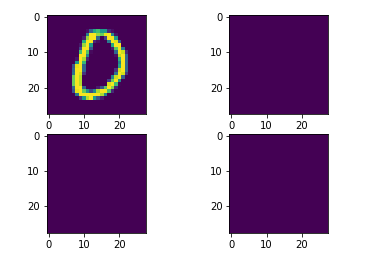# Activation values in ResNet

Hi! I’ve trained a ResNet model and now I’m using it on another dataset and I need access to all the activation values for each neuron for each image. How can i do that?

This is the part of my code that concerns the model:

``````def conv3x3(in_channels, out_channels, stride=1):
return nn.Conv2d(in_channels, out_channels, kernel_size=3,

class ResidualBlock(nn.Module):
def __init__(self, in_channels, out_channels, stride=1, downsample=None):
super(ResidualBlock, self).__init__()
self.conv1 = conv3x3(in_channels, out_channels, stride)
self.bn1 = nn.BatchNorm2d(out_channels)
self.relu = nn.ReLU(inplace=True)
self.conv2 = conv3x3(out_channels, out_channels)
self.bn2 = nn.BatchNorm2d(out_channels)
self.downsample = downsample

def forward(self, x):
residual = x
out = self.conv1(x)
out = self.bn1(out)
out = self.relu(out)
out = self.conv2(out)
out = self.bn2(out)
if self.downsample:
residual = self.downsample(x)
out += residual
out = self.relu(out)
return out

class ResNet(nn.Module):
def __init__(self, block, layers, num_classes=10):
super(ResNet, self).__init__()
self.in_channels = 16
self.conv = conv3x3(3, 16)
self.bn = nn.BatchNorm2d(16)
self.relu = nn.ReLU(inplace=True)
self.layer1 = self.make_layer(block, 16, layers)
self.layer2 = self.make_layer(block, 32, layers, 2)
self.layer3 = self.make_layer(block, 64, layers, 2)
self.avg_pool = nn.AvgPool2d(8)
self.fc = nn.Linear(64, num_classes)

def make_layer(self, block, out_channels, blocks, stride=1):
downsample = None
if (stride != 1) or (self.in_channels != out_channels):
downsample = nn.Sequential(
conv3x3(self.in_channels, out_channels, stride=stride),
nn.BatchNorm2d(out_channels))
layers = []
layers.append(block(self.in_channels, out_channels, stride, downsample))
self.in_channels = out_channels
for i in range(1, blocks):
layers.append(block(out_channels, out_channels))
return nn.Sequential(*layers)

def forward(self, x):
out = self.conv(x)
out = self.bn(out)
out = self.relu(out)
out = self.layer1(out)
out = self.layer2(out)
out = self.layer3(out)
out = self.avg_pool(out)
out = out.view(out.size(0), -1)
out = self.fc(out)
return out

model = ResNet(ResidualBlock, [2, 2, 2]).to(device)

criterion = nn.CrossEntropyLoss()

def update_lr(optimizer, lr):
for param_group in optimizer.param_groups:
param_group['lr'] = lr

model.eval()

correct = 0
total = 0
images = images.to(device)
labels = labels.to(device)
outputs = model(images)
_, predicted = torch.max(outputs.data, 1)
total += labels.size(0)
correct += (predicted == labels).sum().item()

``````

You can use forward hooks as described here to get intermediate activations.

1 Like

how should i change the x = torch.randn(1, 25) part? i don’t know what it’s doing

In my code snippet I’ve used a random input tensor, so you should replace it with your real input data.

1 Like

I was wondering if the part (activation[‘fc2’]) will save all the activations of all the neurons in fc2, how can I access the activation of one single neuron in fc2?

`activation['fc2']` will save the output activation of the `fc2` layer, which you could then index if needed.

for layer 1 = 64 neurons and layer2 = 128 neurons and layer3 = 256 neurons,the shape of activation[‘layer1’] is (100,64,32,32)
what is 100? what are the 32s?

also for activation[‘layer2’] the shape is (100,128,16,16) and for activation['layer3] the shape is (100,256,8,8)

What is the batch size that you used when you printed out these shapes?

batch size is 100. neurons in layer1 are 64. but what are 32 and 32?

1 Like

Hello again! now for a neuron x in the layer `fc2` I’d like to plot the activation value tensor. I want to see all the values for the batch. How do I do this?

You could reshape the activation stored in the forward hook and visualize it e.g. via `matplotlib.pyplot.plot`.

I don’t know how to do it. can you’re please elaborate?

You can use the linked code from my previous reply and use `matplotlib` directly:

``````class MyModel(nn.Module):
def __init__(self):
super(MyModel, self).__init__()
self.cl1 = nn.Linear(25, 60)
self.cl2 = nn.Linear(60, 16)
self.fc1 = nn.Linear(16, 120)
self.fc2 = nn.Linear(120, 84)
self.fc3 = nn.Linear(84, 10)

def forward(self, x):
x = F.relu(self.cl1(x))
x = F.relu(self.cl2(x))
x = F.relu(self.fc1(x))
x = F.relu(self.fc2(x))
x = F.log_softmax(self.fc3(x), dim=1)
return x

activation = {}
def get_activation(name):
def hook(model, input, output):
activation[name] = output.detach()
return hook

model = MyModel()
model.fc2.register_forward_hook(get_activation('fc2'))
x = torch.randn(1, 25)
output = model(x)
print(activation['fc2'])

plt.imshow(activation['fc2'])
``````

It gives me this error:

Invalid shape (1000, 32, 32) for image data.

this tensor is the activation of a neuron x in layer `fc2`.

You cannot plot this tensor via `imshow` as described in your other topic.

how can I make 1000 plots of 32*32?

This should work, but given that you are trying to plot a lot of subplots, you might want to increase the figure size etc.:

``````x = torch.randn(1000, 32, 32)
fig, axs = plt.subplots(20, 50)
for ax, x_ in zip(axs.flatten(), x):
ax.imshow(x_)
``````

I am getting this outputon editing sub plot to 2* 2 only getting first imageAs mentioned in the previous post:

E.g. check the `figsize` argument.

1 Like